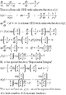# 1st order nonlinear ODE

• all015

#### all015

Can anyone help with the following:

dy/dx = ay / (bx2 +xy )

a,b constants

thanks,

The key is to consider the unknown function x(y) instead of y(x)

#### Attachments

•ODE.JPG
41 KB · Views: 441
The key is to consider the unknown function x(y) instead of y(x)

Hi. Thanks for that. However, I'm not convinced your use of the Ei is correct if you define:

$$Ei(x)=\int_{-\infty}^x \frac{e^t}{t}dt$$

$$d(e^y t)=-\frac{e^y}{y}$$

then integrating from y_0, t_0 to y,t:

$$\int_{\substack{t=t_0 \\ y=y_0}}}^{y,t}d(e^y t)=-\int_{y_0}^y \frac{e^y}{y}dy$$

and get:

$$x(y)=\frac{e^y}{\frac{e^{y_0}}{x_0}-\int_{y_0}^y \frac{e^y}{y}dy}$$

Now, that agrees with numerical calculations but I'm not sure how I could express that in terms of Ei(x) though.

that agrees with numerical calculations but I'm not sure how I could express that in terms of Ei(x) though
Of course, you cannot express that in terms of Ei(x), but in terms of Ei(y).
May be, writing "in terms of" isn't the good wording. What I mean is that Ei(y) is the special function involved in the formula for x(y), as it was shown.
But I never said that Ei(x) is involved in an hypothetical formula for y(x). On the contrary, I said that the analytical inversion of x(y) in order to obtain y(x) is probably utopian with a finite number of elementary functions and even with classical special functions.

thanks guys! - I appreciate the help

Of course, you cannot express that in terms of Ei(x), but in terms of Ei(y).
May be, writing "in terms of" isn't the good wording. What I mean is that Ei(y) is the special function involved in the formula for x(y), as it was shown.
But I never said that Ei(x) is involved in an hypothetical formula for y(x). On the contrary, I said that the analytical inversion of x(y) in order to obtain y(x) is probably utopian with a finite number of elementary functions and even with classical special functions.

Ok, I messed that up. I meant Ei(y) but I see as long as you define Ei(y) appropriately, I see what you mean.

Here's the numerical check of:

$$y'=\frac{y}{x^2+xy},\quad y(1)=1$$

Code:
mysol = NDSolve[{Derivative[y][x] ==
y[x]/(x^2 + x*y[x]), y == 1}, y,
{x, 1, 5}];

p1 = Plot[y[x] /. mysol, {x, 1, 5}];

myx[y_] := Exp[y]/(Exp -
NIntegrate[Exp[u]/u, {u, 1, y}]);

mytable = Table[{myx[y], y},
{y, 1, 1.6, 0.01}];

p2 = ListPlot[mytable, Joined -> True];

Show[{p1, p2}]

Notice how I tabulate the numbers in terms of {x(y),y} to retrieve the inverse numerically. The two plots superimpose nicely.

For me where was no doubt.
Nevertheless its a good idea to check it numerically. Well donne !

Is this a research question, or just homework? If it's serious, I may be able to get somewhere with an analytic (explicit) solution... possibly.